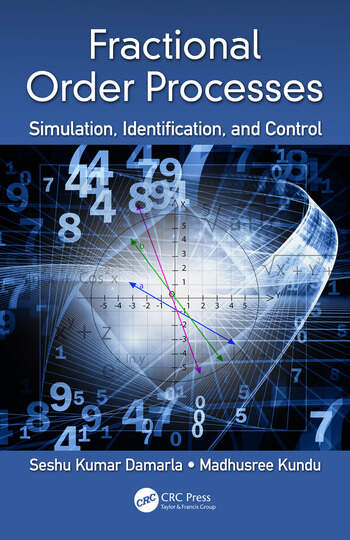# Fractional Order Processes: Simulation, Identification, and Control

## 1st Edition

CRC Press
Published September 4, 2018
Reference - 340 Pages - 49 Color & 9 B/W Illustrations
ISBN 9781138586741 - CAT# K377224

For Instructors Request Inspection Copy

was \$139.95

USD\$111.96

SAVE ~\$27.99

FREE Standard Shipping!

## Preview

### Summary

The book presents efficient numerical methods for simulation and analysis of physical processes exhibiting fractional order (FO) dynamics. The book introduces FO system identification method to estimate parameters of a mathematical model under consideration from experimental or simulated data. A simple tuning technique, which aims to produce a robust FO PID controller exhibiting iso-damping property during re-parameterization of a plant, is devised in the book. A new numerical method to find an equivalent finite dimensional integer order system for an infinite dimensional FO system is developed in the book. The book also introduces a numerical method to solve FO optimal control problems.

Key features

• Proposes generalized triangular function operational matrices.
• Shows significant applications of triangular orthogonal functions as well as triangular strip operational matrices in simulation, identification and control of fractional order processes.
• Provides numerical methods for simulation of physical problems involving different types of weakly singular integral equations, Abel’s integral equation, fractional order integro-differential equations, fractional order differential and differential-algebraic equations, and fractional order partial differential equations.
• Suggests alternative way to do numerical computation of fractional order signals and systems and control.
• Provides source codes developed in MATLAB for each chapter, allowing the interested reader to take advantage of these codes for broadening and enhancing the scope of the book itself and developing new results.

#### Instructors

We provide complimentary e-inspection copies of primary textbooks to instructors considering our books for course adoption.